# How Results in the Evaluation Forms Are Calculated

This article explains why weightings in evaluation forms play an important role and how they are calculated.

Personio allows you to manage the feedback on applicants in an objective and structured way with the use of evaluation forms. An evaluation form consists of one or more sections which you can use to query user-defined criteria on the applicant. A section may consist of a title as well as text and/or scale fields. Scales play an important role when evaluation forms are used.

## Weighting of Scales

Scales consist of descriptive text (title), the labels for the lowest and highest scores, and a weighting. A further field, "Info for evaluator", is available to add a comment.

The following image shows scales as displayed from an interviewer's perspective:The descriptive text (e.g. "Technical competency") and the labels for the lowest and highest scores are directly visible. The "Info for evaluator" can be found in the information symbol ("i") next to the descriptive text. The weighting of the scales is not visible to the evaluator.

If your scales are to be weighted equally, a weighting of -1- has to be selected for each.

Choose weightings between 0 and 1 if you want to assign varying levels of importance to individual scales. The result is calculated irrespectively of whether the individual weightings add up to -1- or not. See an example below with several scales per section and weightings of less than -1-.

Important: Please use a point for decimal values (instead of a comma).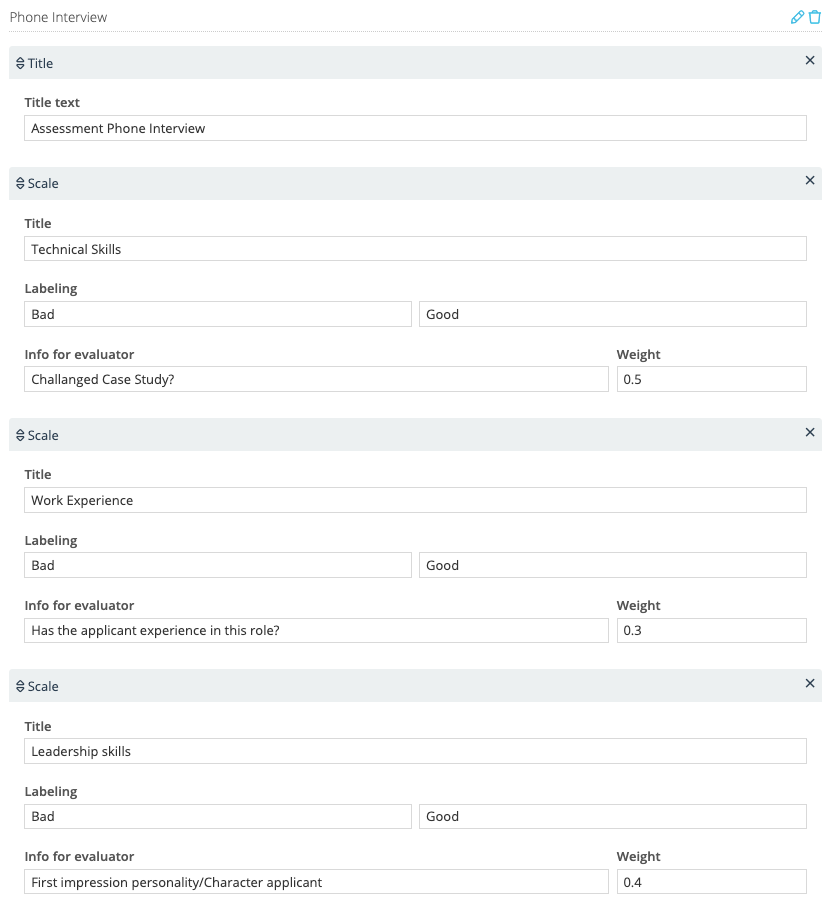## Calculation of Scales

Calculation logic

Multiple scores within scales are combined in order to come up with the overall score for the evaluation form. The calculation logic is explained in the following example:

Three scales, for each of which a score is given:

A (Technical competence)

B (Professional experience)

Three weightings:

X, Y and Z for the three scales

The result is calculated using the following formula:

[(Score Scale A x Weighting X) + (Score Scale B x Weighting Y) + (Score Scale C x Weighting Z)] / (Weighting X + Weighting Y + Weighting Z)

The results from this calculation are rounded up or down according common rounding rules.

Concrete examples

Scores with a weighting of -1- each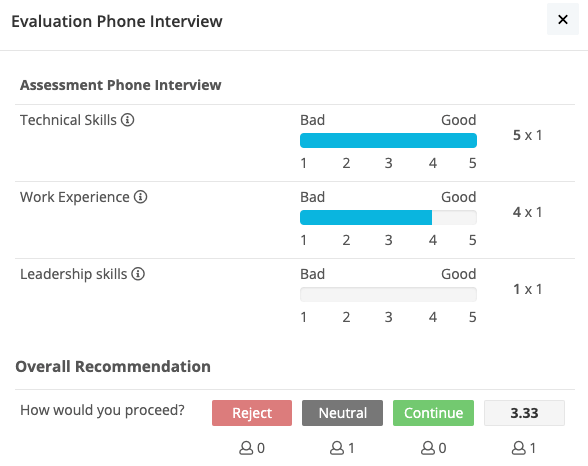The following is the calculation:

[(5x1) + (4x1) + (1x1)] / (1+1+1)

= 10 / 3

= 3.33

The overview shows the value rounded to one decimal place: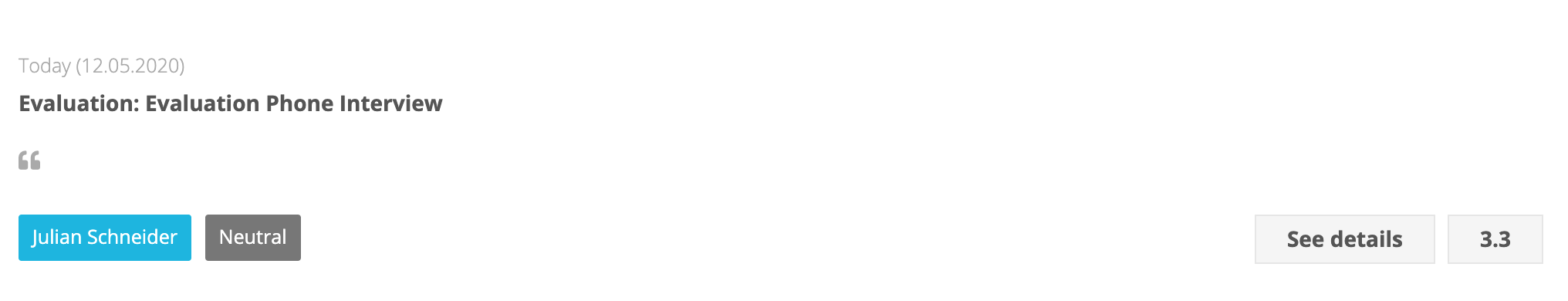Scores with a weighting of below -1-The following is the calculation:

[(5x0.5) + (4x0.3) + (1x0.4)] / (0.5+0.3+0.4)

= 4.1 / 1.2

= 3.42

The overview shows the value rounded to one decimal place: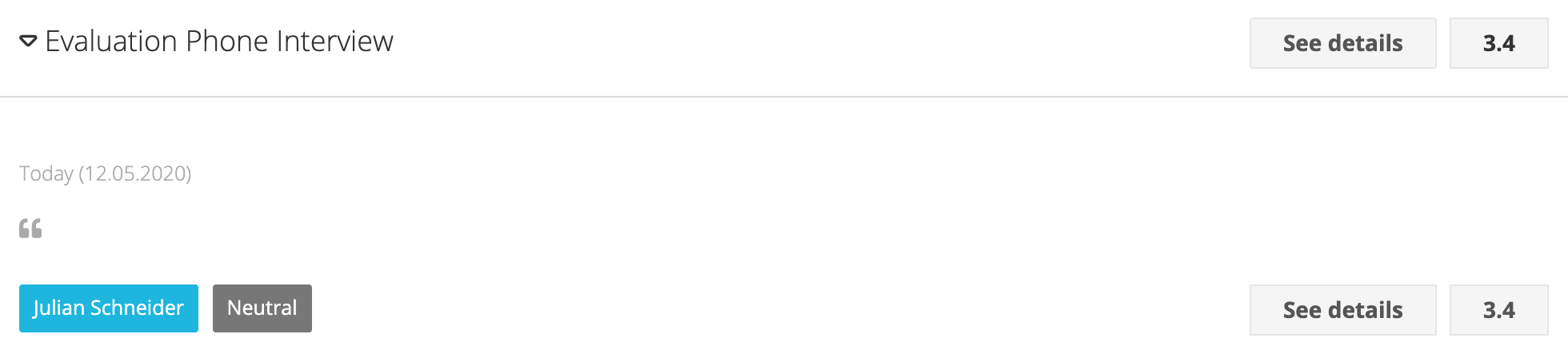## Calculation of the Overall Score

If multiple evaluations are completed for an applicant, the overall score will be based on their results: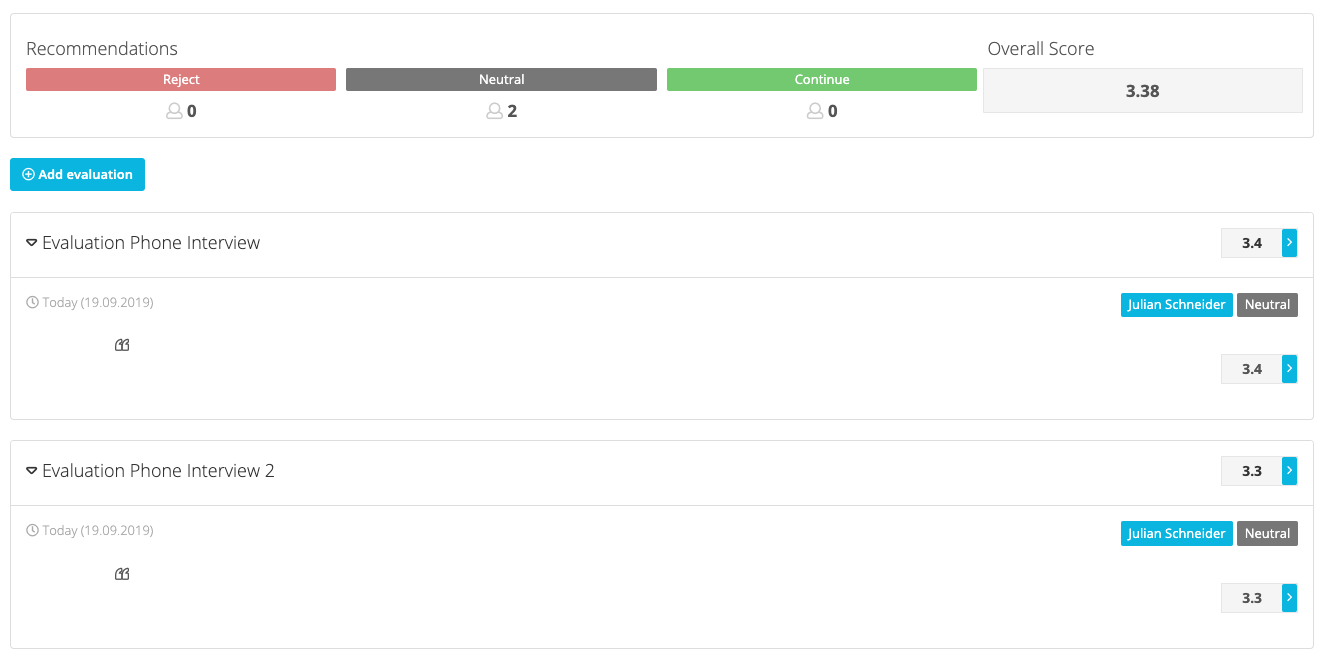The following is the calculation:

[Result A (not rounded) + Result B (not rounded)] / Number of results

(3.42 + 3.33) / 2

= 6.75 / 2

= 3.375

= rounded 3.38

The overall score is thereby rounded to two decimal places.